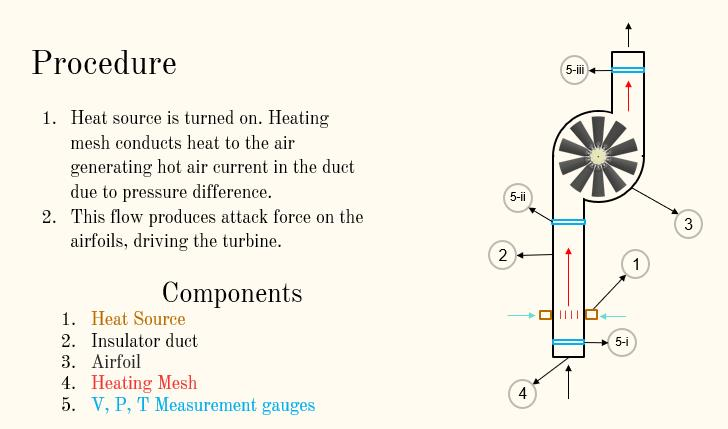## Fluids

Topics relate to Fluent, CFX, Turbogrid and more

•Muhammad Abdul Hadi
Subscriber

Can we study thermodynamic properties of fluid and the work outputs in ANSYS. I'm working on a thermodynamics course project in which we generate Convection Currents that are going to drive a turbine (or propeller/airfoils). I want to study the thermodynamics properties, TS and PV diagrams and work outputs. It's very difficult to fabricate an apparatus to carry out the experiment. Therefore, I'm going for ANSYS (if it is applicable to the stated problem).

•peteroznewman
Subscriber

A simulation of the physics you describe is possible in FLUENT.  A good way to start is to imagine the simplest geometry possible that captures everything you want to see in the results.  You might want more complicated geometry to achieve higher efficiencies, but start with simple geometry to get an initial model working. If you reply with a sketch of that simple geometry and the boundary conditions on that geometry, I am sure you will get some help to make that model run.

•Muhammad Abdul Hadi
SubscriberThis is the longitudinal section of the system we want to simulate.

It is placed in open environment i. e. room temperature and pressure. We want to study the behavior at the points 5 (i, ii, iii). and to develop this process into cyclic (thermodynamically). Can you help us simulating this process.

•Muhammad Abdul Hadi
Subscriber

and can we obtain graphs (PV, TS, HS etc.) for a given cycle in ANSYS.

•Muhammad Abdul Hadi
Subscriber

We also want to find the output work at airfoils

•peteroznewman
Subscriber

I know how to model structures. I am here to learn how to model thermal and fluid systems. Outlined below is my understanding of how to go about this but I am looking for confirmation or corrections from more knowledgeable FLUENT modelers.

I found a good explanation of the graphs you mentioned.

I assume you want the steady-state condition to draw these graphs. A transient model simulates the development of flow from the time the switch was turned on. An example of a turbine is shown in the video below.  There may be a way to build a steady-state model that is simpler, so I will wait for others to comment.

2D PLANAR SYMMETRY
Two geometries could be simulated here: 2D and 3D.  If you take your diagram above and extrude it a long distance into the page, that is a 2D model, and the end walls are so distant as to be irrelevant. Call this shape a channel. If the inlet and outlet sections were pipes and there was a cylindrical chamber for the turbine, that is a 3D model.  If that diagram was extruded to make a square duct, that would still be a 3D model because the end walls are near enough to affect the flow. I recommend you first build a 2D model as it is quicker and easier.

IDEALIZE HEATING MESH
In a 3D model, you could draw individual heating wires crossing the duct to make a mesh and assign a fixed heat flux to each wire. In a 2D model, you won't be able to have a mesh. You can use a set of parallel wires and draw the cross-section of the wire diameter as a pattern of circles across the width of the channel. The pressure loss of the air flowing around the wires will be calculated and the heat transfer through the boundary layer will be calculated in the solution.

6DOF MESH
I believe you will need what is called the 6 DOF mesh method in order to have the fluid turn the turbine. Watch Raef's tutorial for how to use a dynamic mesh in FLUENT. This is a transient model example so you will have to find out how to use this for steady-state. One of the inputs you will have to provide is the polar mass moment of inertia of the turbine blade. You can calculate that in a CAD system. Another input is the torque required to turn the generator.